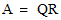# NMath User's Guide

21.2 Creating Ordinary Least Squares Objects (.NET, C#, CSharp, VB, Visual Basic, F#)

NMath provides ordinary least squares classes for four datatypes: single- and double-precision floating point numbers, and single- and double-precision complex numbers. The classnames are shown in Table 18.

 Least Squares Method Classes Cholesky QR Decomposition SVD

Instances of the least squares classes are constructed from general matrices of the appropriate datatype. For example, this code creates a FloatCholeskyLeastSq from a FloatMatrix:

Code Example – C# least squares

```FloatMatrix A = new FloatMatrix( "4x2[ 1 0  0 1  0 0  0 0 ]" );
```
```FloatCholeskyLeastSq lsq = new FloatCholeskyLeastSq( A );

```

Code Example – VB least squares

```Dim A As New FloatMatrix("4x2[ 1 0 0 1 0 0 0 0]")
```
```Dim LSQ As New FloatCholeskyLeastSq(A)
```

QR and SVD least squares classes also provide constructor overloads that accept a tolerance value. The specified tolerance is used in computing the numerical rank of the matrix. For example, ifis the QR factorization of a matrix A, then elements on the main diagonal of R are considered to be zero if their absolute value is less than or equal to the tolerance. Similarly, in singular value decomposition, all singular values of the matrix A less than the tolerance are set to zero. Thus, this code sets all singular values less than 10-13 to zero:

Code Example – C# least squares

```DoubleMatrix A = new DoubleMatrix( "4x2[ 1 0  0 1  0 0  0 0 ]" );
```
```DoubleSVDLeastSq lsq = new DoubleSVDLeastSq( A, 1e-13 );
```

Code Example – VB least squares

```Dim A As New DoubleMatrix( "4x2[1 0 0 1 0 0 0 0 ]" )
```
```Dim LSQ As New DoubleSVDLeastSq( A, "1e-13" )
```

Top

Top